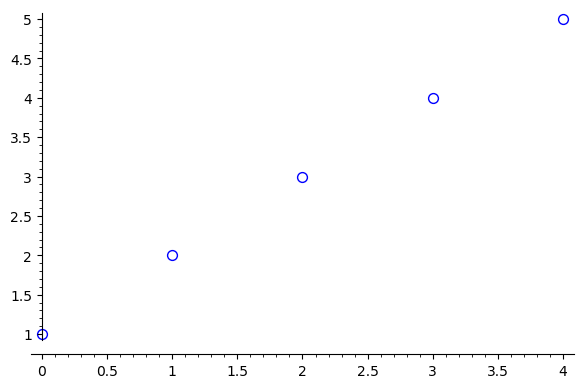# R plot in sage not showing

Hello, I'm trying to use r.plot() in Sage9.0, but when I do I only get null device 1 as a output in Jupyter. Can someone please tell me what exactly I'm doing wrong here? I'm on ubuntu18.04.

x <- r([1,2,3,4,5]) y <- r([2,3,4,5,2]) r.plot(x)

null device 1

edit retag close merge delete

Sort by » oldest newest most voted

Hello, @Alex89! The problem seems to be Jupyter not being able to handle R's plot command interactively. I tried the following:

x = r([1,2,3,4,5])
y = r([2,3,4,5,2])
r.plot(x)


I got the null device 1 message, but my home folder (where I opened the Jupyter Notebook) now has a file called Rplot001.png. So, Jupyter is delegating the plotting to another output device (PNG in this case).

However, I have an alternative for you:

x = r([1,2,3,4,5])
y = r([2,3,4,5,2])
list_plot(x.sage(), size=50)


The first two commands create R objects x and y. You can work with them in R language. For example,

print(~x)
print(x.mean())
print(x.var())


will print

 1.0000000 0.5000000 0.3333333 0.2500000 0.2000000
 3
 2.5


Anyway, going back to the first piece of code, if you want to create an interactive plot in Jupyter, you should do it through Sage. If you see the last line, I first converted the R object x to a Sage object with x.sage(), and then I used the list_plot() command from Sage, which creates a plot similar to R's plot() command.

If you want a more R-looking plot, you can play with some options. For example,

list_plot(x.sage(), size=50, faceted=True, color='white', markeredgecolor='blue')


producesI hope this helps!

EDIT (for completeness): As you can see on the figure, Sage's list_plot assumes the default domain for the plot is ${0, 1, 2, 3, \ldots}$, while R's plot assumes it to be ${1, 2, 3, \ldots}$. If you want to specify a different domain, you can check this related question. In particular, you can do

xs = x.sage()
xx = [(i+1,xs[i]) for i in range(len(xs))]
list_plot(xx, size=50, faceted=True, color='white', markeredgecolor='blue')


to use R's default domain, ${1, 2, 3, \ldots}$.

more

Thanks for this one. Wish they made R more interactive in SageMath.

The version of rpy2 sage is using is very much outdated. See a proposal for upgrade at ticket #29441.